# Segment of a Circle

Segment of a Circle
Go back to  'Circles'

In this lesson, we will expand our knowledge about cirles by learning about the segment of a circle, which will include its mathematical definition, area of segment, types of segments in a circle, theorems based on segment of a circle and related real life word problems and solutions.

A segment of a circle is the region enclosed by a chord and an arc so formed touching the end points of the chord.

In the simulation given below, drag the end point of the chord to form segment of a circle.

Isn't it interesting? Let's explore further.

## What is Segment of a Circle?

Cersie and her brother had a chocolate pie that they had to distribute equally among themselves. But, Cersei was clever to cut it in such a way that she got the larger slice.She did not cut it through the centre of the circular pie, but rather she cut it through another line known as chord of a circle to create segments of pie.

Now let us look at the definition of segment of a circle.

### Definition

A segment of a circle is the region enclosed by a chord and an arc so formed touching the end points of the chord.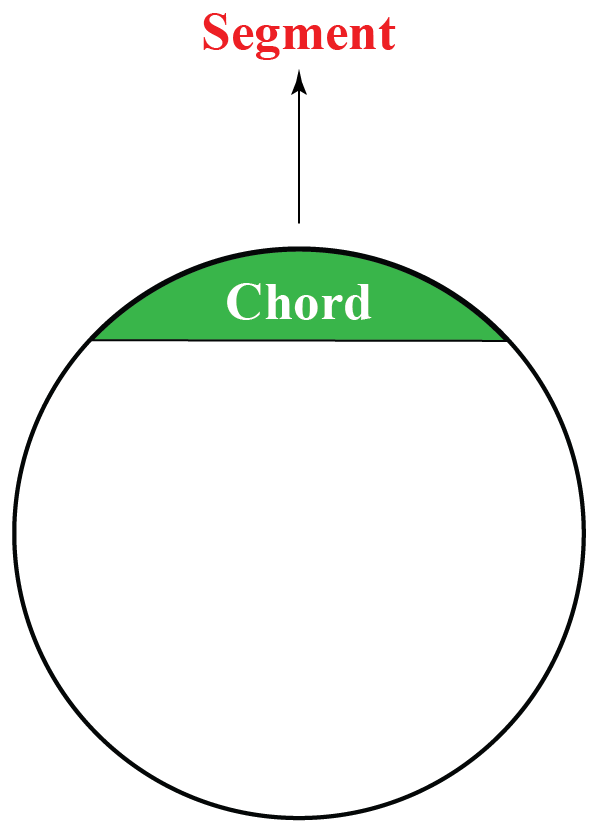If we break a circle into two parts by a straight line touching the circumference of the circle, those two parts are called segments of the circle.

## What is the Area of a Segment?

Area of a segment is the area enclosed between the chord and the minor or major arc of the circle.

We find the area of a segment with the help of central angle formed by the chord and radius of the circle (denoted as $$\theta$$ in the figure given below)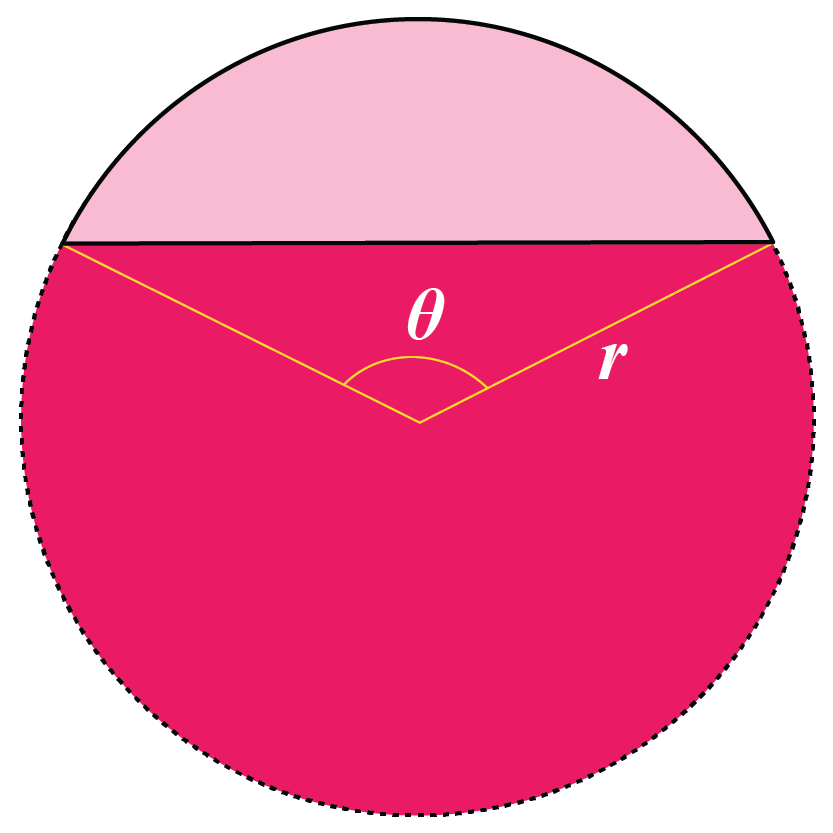Area of the Segment can be found by taking the differance of the Area of the sector and the Area of the triangle enclosed in it. Or else, it can be found by subtracting the area of the other given segment (major or minor) from the area of the circle.

## Types of Segment in a Circle?

According to the area enclosed by the segments, it can be classified into two types: major segment and minor segment. The major segment covers a larger portion of the circle, while the minor segment covers a smaller portion of the circle.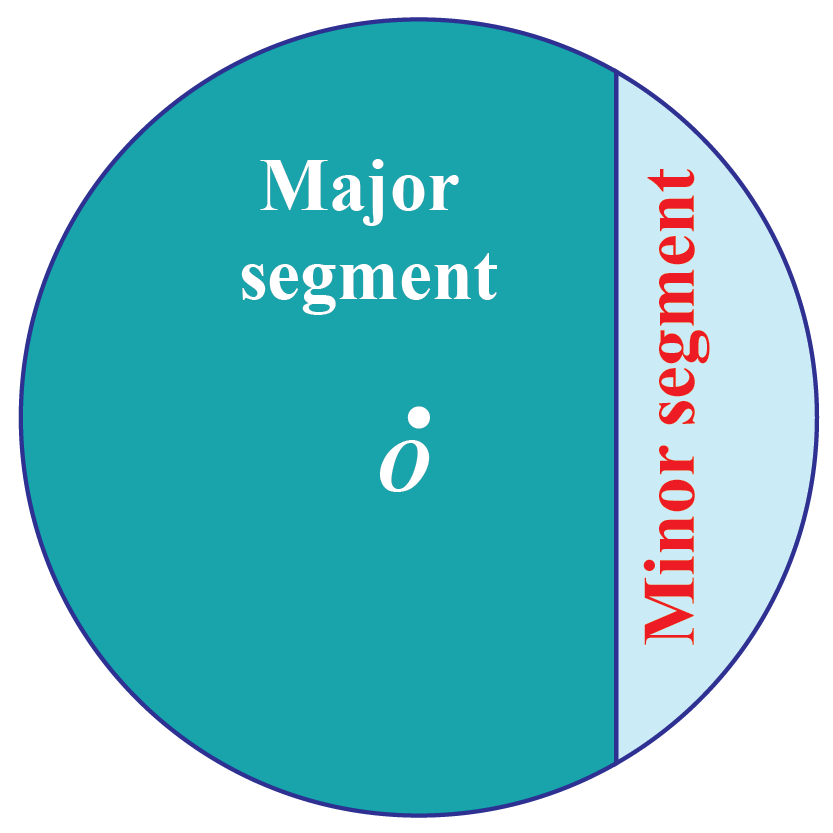## Area Formula of Segment of a Circle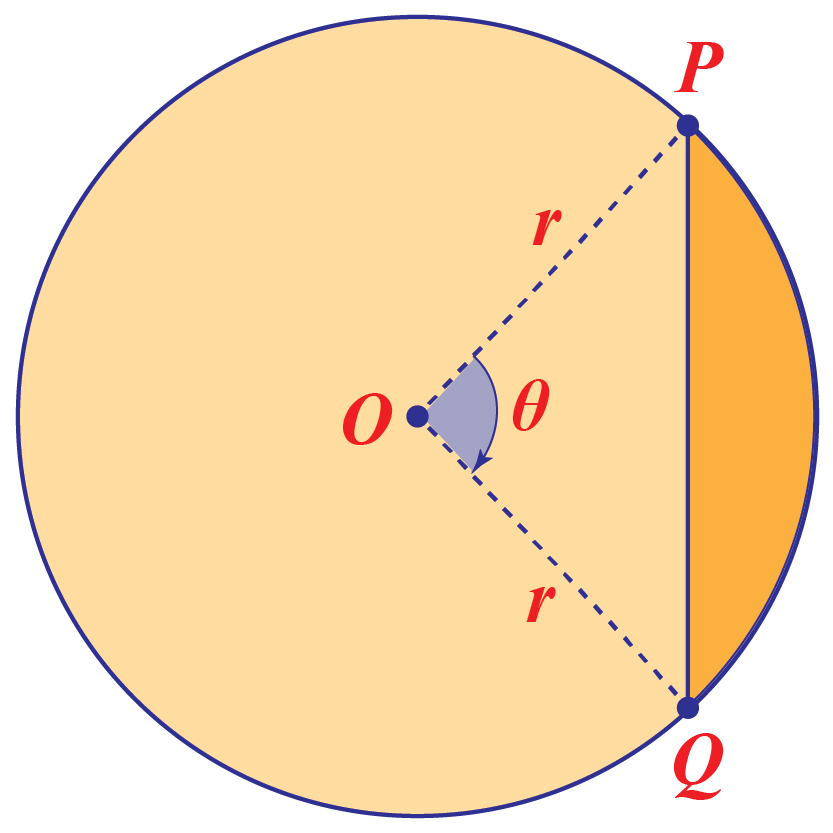$$A= \frac{1}{2} \times \ r^2 \ (\theta-\sin\ \theta)$$
 Formula to be used to find Area of Segment Area of a Segment in Radians $$A= \frac{1}{2} \times \ r^2 \ (\theta-\sin\ \theta)$$ Area of a Segment in Degrees $$A= \frac{1}{2} \times \ r^2 \ [\frac{\pi}{180^o}(\theta-\sin\ \theta)]$$

### Segment of a Circle Calculator

In the simulation below, drag points P and Q to change the radius and central angle and carefully observe the area of segment so formed.

## Theorems on Segment of a Circle

Mainly, there are two theorems based on the segment of a Circle.

1. Angles in the same segment theorem
2. Alternate segment theorem

### Angles in the Same Segment Theorem

It states that angles formed in the same segment of a circle are always equal.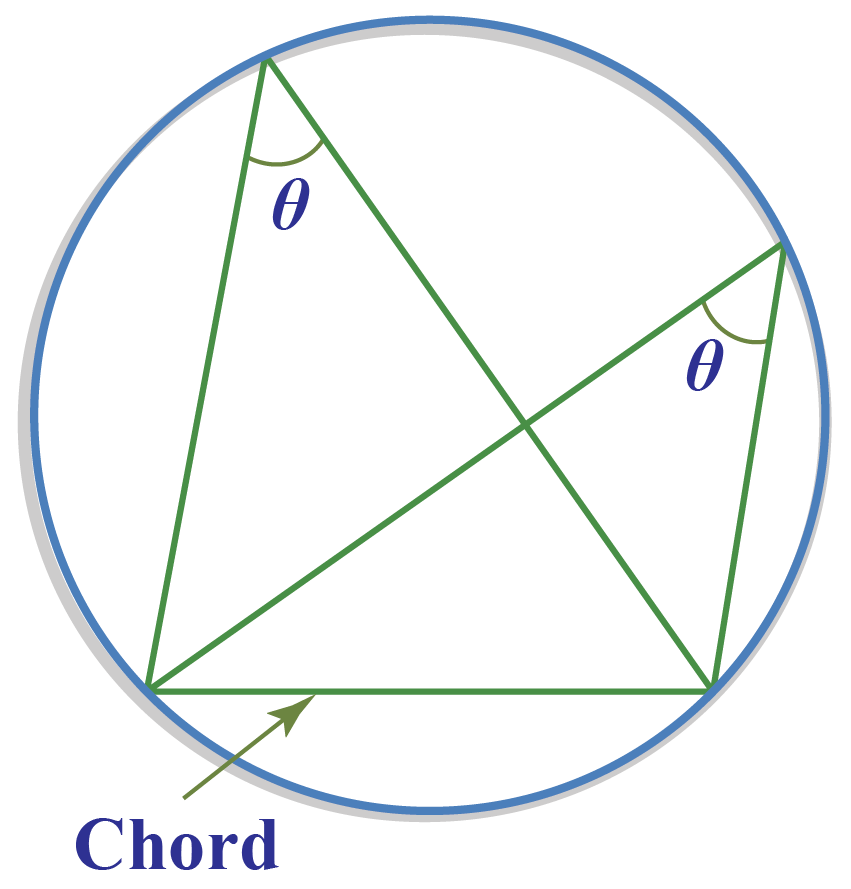### Alternate Segment Theorem

This theorem states that the angle formed by the tangent and the chord at the point of contact is equal to the angle formed in the alternate segment on the circumference of the circle through the end points of the chord.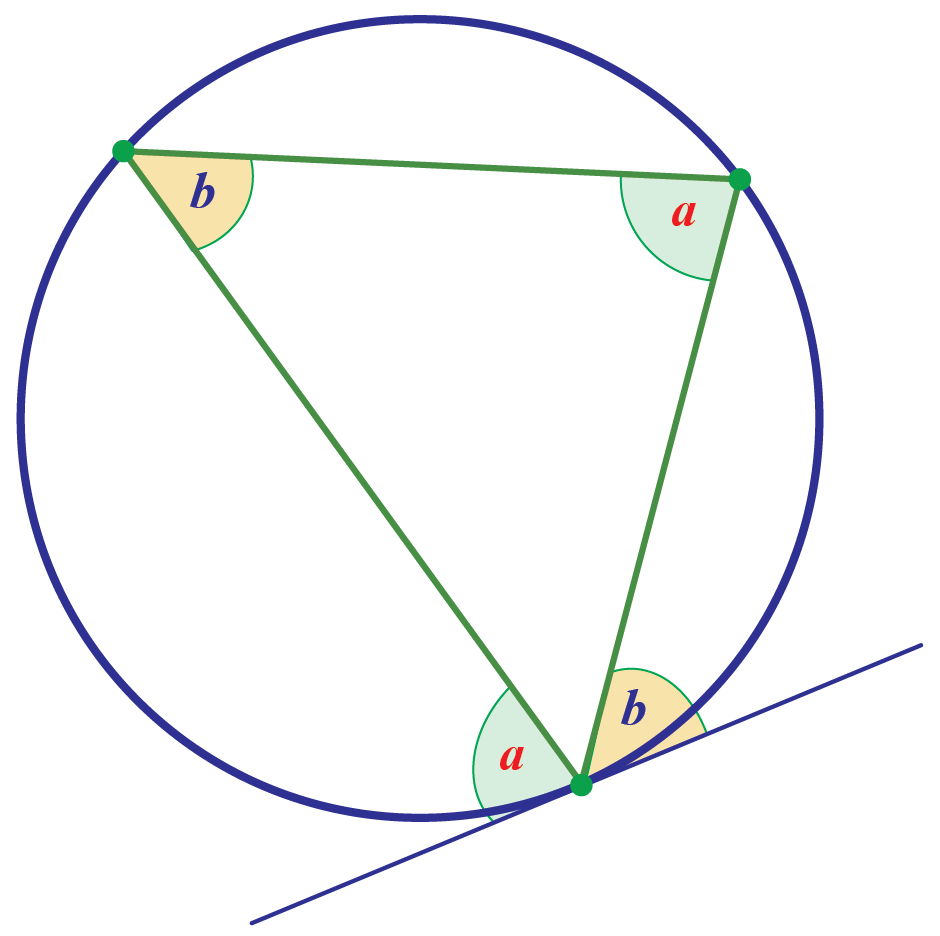More Important Topics
Numbers
Algebra
Geometry
Measurement
Money
Data
Trigonometry
Calculus